IMO Shortlist 2009 problem G7

Kvaliteta:
Avg: 0,0
Težina:
Avg: 9,0
Let$ABC$ be a triangle with incenter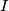$I$ and let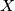$X$,$Y$ and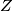$Z$ be the incenters of the triangles$BIC$,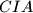$CIA$ and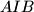$AIB$, respectively. Let the triangle$XYZ$ be equilateral. Prove that$ABC$ is equilateral too.

Proposed by Mirsaleh Bahavarnia, Iran
Izvor: Međunarodna matematička olimpijada, shortlist 2009